• Nota de Estudos
• Rever Tópicos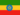Henock A. 0 0 done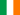Rachel N. 0 0 Describing patterns in time series dataRachel N. 0 0 A time series is a set of values (y1,y2,y3... ... etc.) observed at times t1,t2,t3... ...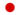Isamu N. 0 0 trend, seasonal, cyclic and ramdom.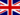Paul B. 1 0 Not sure what the video is about ?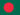Jahidul I. 0 0 Describing patterns in time series data A time series is a set of values (y1,y2,y3... ... etc.) observed at times t1,t2,t3... ... A time series can be classified as having one or more of the following patterns: (1) trend (2) seasonal (3) cyclic (4) random View the video of patterns in pathways and answer the questions on time series data. Your questions and answers can bIn the following topics, we will first review techniques used to identify patterns in time series data (such as smoothing and curve fitting techniques and autocorrelations), then we will introduce a general class of models that can be used to represent time series data and generate predictions (autoregressive and moving average models). Finally, we will review some simple but commonly used modeling and forecasting techniques based on linear regression. For more information see the topics below. General Introduction Two Main Goals Identifying Patterns in Time Series Data Systematic pattern and random noise Two general aspects of time series patterns Trend Analysis Analysis of Seasonality ARIMA (Box & Jenkins) and Autocorrelations General Introduction Two Common Processes ARIMA Methodology Identification Phase Parameter Estimation Evaluation of the Model Interrupted Time Series Exponential Smoothing General Introduction Simple Exponential Smoothing Choosing the Best Value for Parameter a (alpha) Indices of Lack of Fit (Error) Seasonal and Non-seasonal Models With or Without Trend Seasonal Decomposition (Census I) General Introduction Computationse printed.Jahidul I. 0 0 what is Periodogram ?
• Text Version### Você recebeu uma nova notificação

Clique aqui para visualizar todos eles